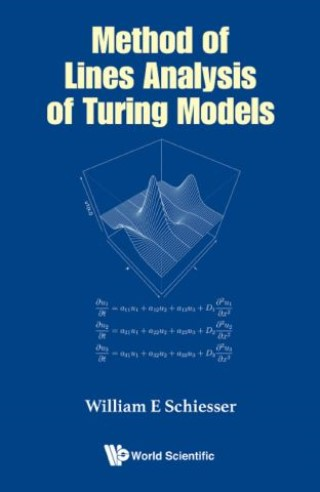## Method Of Lines Analysis Of Turing Models• Description
• Author
• Info
• Reviews

### Description

This book is directed toward the numerical integration (solution) of a system of partial differential equations (PDEs) that describes a combination of chemical reaction and diffusion, that is, reaction-diffusion PDEs. The particular form of the PDEs corresponds to a system discussed by Alan Turing and is therefore termed a Turing model.

Specifically, Turing considered how a reaction-diffusion system can be formulated that does not have the usual smoothing properties of a diffusion (dispersion) system, and can, in fact, develop a spatial variation that might be interpreted as a form of morphogenesis, so he termed the chemicals as morphogens.

Turing alluded to the important impact computers would have in the study of a morphogenic PDE system, but at the time (1952), computers were still not readily available. Therefore, his paper is based on analytical methods. Although computers have since been applied to Turing models, computer-based analysis is still not facilitated by a discussion of numerical algorithms and a readily available system of computer routines.

The intent of this book is to provide a basic discussion of numerical methods and associated computer routines for reaction-diffusion systems of varying form. The presentation has a minimum of formal mathematics. Rather, the presentation is in terms of detailed examples, presented at an introductory level. This format should assist readers who are interested in developing computer-based analysis for reaction-diffusion PDE systems without having to first study numerical methods and computer programming (coding).

The numerical examples are discussed in terms of: (1) numerical integration of the PDEs to demonstrate the spatiotemporal features of the solutions and (2) a numerical eigenvalue analysis that corroborates the observed temporal variation of the solutions. The resulting temporal variation of the 2D and 3D plots demonstrates how the solutions evolve dynamically, including oscillatory long-term behavior.

In all of the examples, routines in R are presented and discussed in detail. The routines are available through a download link so that the reader can execute the PDE models to reproduce the reported solutions, then experiment with the models, including extensions and application to alternative models.

Contents:
• One Dimensional PDEs Introduction
• Eigenvalue Analysis
• Nonlinear Models
• Alternate Coordinate Systems
• Two Dimensional PDEs

Readership: Biologists, medical researchers and clinicians, biophysicists, biochemists, biomathematicians, anthropologists, engineers (biomedical, electrical, chemical).
Turing Morphogenesis Equations;Partial Differential Equations;Method of Lines0